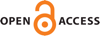Download this articleFor screen For printingRecent IssuesThe Journal About the Journal Editorial Board Editors’ Interests Subscriptions Submission Guidelines Submission Form Policies for Authors Ethics Statement ISSN: 1948-206X (e-only) ISSN: 2157-5045 (print) Author Index To Appear Other MSP JournalsPartial regularity for Navier–Stokes and liquid crystals inequalities without maximum principle

### Gabriel S. Koch

Vol. 16 (2023), No. 7, 1701–1744##### Abstract

In 1985, V. Scheffer discussed partial regularity results for what he called solutions to the Navier–Stokes inequality. These maps essentially satisfy the incompressibility condition as well as the local and global energy inequalities and the pressure equation which may be derived formally from the Navier–Stokes system of equations, but they are not required to satisfy the Navier–Stokes system itself.

We extend this notion to a system considered by Fang-Hua Lin and Chun Liu in the mid 1990s related to models of the flow of nematic liquid crystals, which include the Navier–Stokes system when the director field $d$ is taken to be zero. In addition to an extended Navier–Stokes system, the Lin–Liu model includes a further parabolic system which implies an a priori maximum principle for $d$ which they use to establish partial regularity (specifically, ${\mathsc{𝒫}}^{1}\left(\mathsc{𝒮}\right)=0$) of solutions.

For the analogous inequality one loses this maximum principle, but here we nonetheless establish the partial regularity result ${\mathsc{𝒫}}^{9∕2+\delta }\left(\mathsc{𝒮}\right)=0$, so that in particular the putative singular set $\mathsc{𝒮}$ has space- time Lebesgue measure zero. Under an additional assumption on $d$ for any fixed value of a certain parameter $\sigma \in \left(5,6\right)$ — which for $\sigma =6$ reduces precisely to the boundedness of $d$ used by Lin and Liu — we obtain the same partial regularity (${\mathsc{𝒫}}^{1}\left(\mathsc{𝒮}\right)=0$) as do Lin and Liu. In particular, we recover the partial regularity result (${\mathsc{𝒫}}^{1}\left(\mathsc{𝒮}\right)=0$) of Caffarelli–Kohn–Nirenberg  for suitable weak solutions of the Navier–Stokes system, and we verify Scheffer’s assertion that the same holds for solutions of the weaker inequality as well.

We remark that the proofs of partial regularity both here and in the work of Lin and Liu largely follow the proof in Caffarelli–Kohn–Nirenberg, which in turn used many ideas from an earlier work of Scheffer .

##### Keywords
Navier–Stokes, liquid crystals, partial regularity
##### Mathematical Subject Classification
Primary: 35A21, 35B65, 35D30, 35G50, 35Q35
##### Milestones